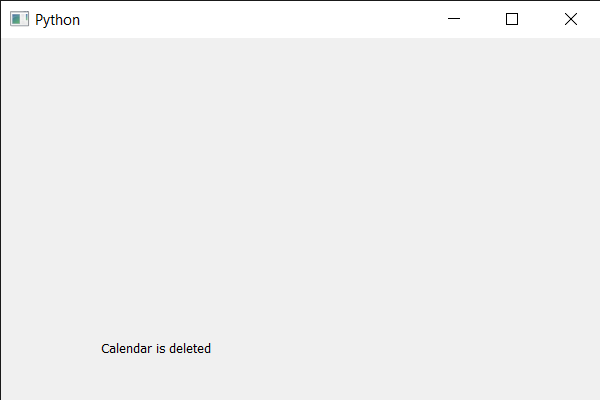# PyQt5 QCalendarWidget – Removing memory reference

• Last Updated : 04 Jul, 2021

In this article, we will see how we can get delete the reference of the QCalendarWidget. Removing the last Python reference of the calendar object won’t be enough to fully clean up, because there could still be a reference held on the Qt side. In general, Qt tends not to implicitly delete objects. So there may be a need to take steps to delete them explicitly if memory usage is a concern.

In order to do this we will use deleteLater method with the QCalendarWidget object.
Syntax : calendar.deleteLater()
Argument : It takes no argument
Return : It returns None

Below is the implementation

## Python3

 `# importing libraries``from` `PyQt5.QtWidgets ``import` `*``from` `PyQt5 ``import` `QtCore, QtGui``from` `PyQt5.QtGui ``import` `*``from` `PyQt5.QtCore ``import` `*``import` `sys`  `class` `Window(QMainWindow):` `    ``def` `__init__(``self``):``        ``super``().__init__()` `        ``# setting title``        ``self``.setWindowTitle(``"Python "``)` `        ``# setting geometry``        ``self``.setGeometry(``100``, ``100``, ``600``, ``400``)` `        ``# calling method``        ``self``.UiComponents()` `        ``# showing all the widgets``        ``self``.show()` `    ``# method for components``    ``def` `UiComponents(``self``):` `        ``# creating a QCalendarWidget object``        ``calendar ``=` `QCalendarWidget(``self``)` `        ``# setting geometry to the calendar``        ``calendar.setGeometry(``10``, ``10``, ``400``, ``250``)` `        ``# deleting the memory reference explicitly``        ``calendar.deleteLater()` `        ``# creating a label``        ``label ``=` `QLabel(``self``)` `        ``# setting geometry to the label``        ``label.setGeometry(``100``, ``280``, ``250``, ``60``)` `        ``# making label multi line``        ``label.setWordWrap(``True``)` `        ``# setting text to the label``        ``label.setText(``"Calendar is deleted"``)` `# create pyqt5 app``App ``=` `QApplication(sys.argv)` `# create the instance of our Window``window ``=` `Window()` `# start the app``sys.exit(App.``exec``())`

Output :My Personal Notes arrow_drop_up